# AP Calculus BC : P-Series

## Example Questions

### Example Question #1 : P Series

One of the following infinite series CONVERGES. Which is it?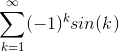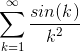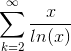None of the others converge.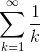Explanation:converges due to the comparison test.

We start with the equation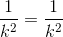. Since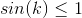for all values of k, we can multiply both side of the equation by the inequality and get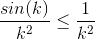for all values of k. Since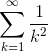is a convergent p-series with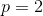hence also converges by the comparison test.

### Example Question #2 : P Series

Determine the nature of convergence of the series having the general term: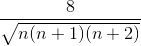The series is convergent.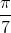The series is divergent.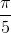The series is convergent.

Explanation:

We will use the Limit Comparison Test to establish this result.

We need to note that the following limit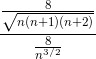goes to 1 as n goes to infinity.

Therefore the series have the same nature. They either converge or diverge at the same time.

We will focus on the series: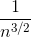.

We know that this series is convergent because it is a p-series. (Remember that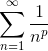converges if p>1 and we have p=3/2 which is greater that one in this case)

By the Limit Comparison Test, we deduce that the series is convergent, and that is what we needed to show.

### Example Question #3 : P Series

Determine if the series converges or diverges. You do not need to find the sum.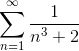Conditionally converges.

There is not enough information to decide convergence.

Diverges

Neither converges nor diverges.

Converges

Converges

Explanation:

We can compare this to the series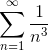which we know converges by the p-series test.

To figure this out, let's first compare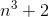to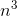. For any number n,will be larger than.

There is a rule in math that if you take the reciprocal of each term in an inequality, you are allowed to flip the signs.

Thus,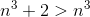turns into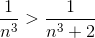.

And so, because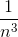converges, thus our series also converges.

### Example Question #2998 : Calculus Ii

Which of the following tests will help determine whether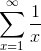is convergent or divergent, and why?Nth Term Test: The series diverge because the limit asgoes to infinity is zero.

P-Series Test: The summation converges since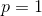.

Root Test: Since the limit asapproaches to infinity is zero, the series is convergent.Divergence Test: Since limit of the series approaches zero, the series must converge.

Integral Test: The improper integral determines that the harmonic series diverge.

Integral Test: The improper integral determines that the harmonic series diverge.

Explanation:

The seriesis a harmonic series.

The Nth term test and the Divergent test may not be used to determine whether this series converges, since this is a special case.  The root test also does not apply in this scenario.

According the the P-series Test,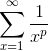must converge only if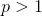.  Therefore this could be a valid test, but a wrong definition as the answer choice since the series diverge for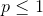.

This leaves us with the Integral Test.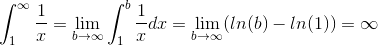Since the improper integral diverges, so does the series.

### Example Question #4 : P Series

Does the series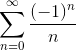converge conditionally, absolutely, or diverge?

Cannot tell with the given information.

Converge Absolutely.

Does not exist.

Converge Conditionally.

Diverges.

Converge Conditionally.

Explanation:

The series converges conditionally.

The absolute values of the series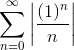is a divergent p-series with.

However, the the limit of the sequence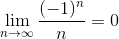and it is a decreasing sequence.

Therefore, by the alternating series test, the series converges conditionally.

### Example Question #5 : P Series

True or False, a-series cannot be tested conclusively using the ratio test.

False

True

True

Explanation:

We cannot test for convergence of a-series using the ratio test. Observe,

For the series,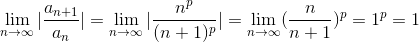.

Since this limit isregardless of the value for, the ratio test is inconclusive.